# Transformer,three phase:Wye/Delta or Y/∆ Connection and Delta/Wye or ∆/Y Connection.

## Wye/Delta or Y/∆  Connection

The main use of this connection is at the substation end of the transmission line where the voltage is to be stepped down. The primary winding is Y-connected with grounded neutral as shown in Fig. 33.6. The ratio between the secondary and primary line voltage is 1/ 3 times the transformation ratio of each trans- former. There is a 30° shift between the primary and secondary line voltages which means that a Y – D transformer bank cannot be paralleled with either a Y Y or a D – D bank. Also, third harmonic currents flows in the D to provide a sinusoidal flux.

## Delta/Wye or ∆/Y Connection

This connection is generally employed where it is necessary to step up the voltage as for example, at the beginning of high tension transmission system. The connection is shown in Fig. 33.7. The neutral of the secondary is grounded for providing 3-phase 4-wire service. In recent years, this connection has gained considerable popularity because it can be used to serve both the 3-phase power equipment and single-phase lighting circuits.

This connection is not open to the objection of a floating neutral and voltage distortion because the existence of a D-connection allows a path for the third-harmonic currents. It would be observed that the primary and secondary line voltages and line currents are out of phase with each other by 30°. Because of this 30° shift, it is impossible to parallel such a bank with a D – D or Y Y bank of transformers even though the voltage ratios are correctly adjusted. The ratio of secondary to primary voltage is 3 times the transformation ratio of each transformer.

Example 33.1. A 3-phase, 50-Hz transformer has a delta-connected primary and star-connected secondary, the line voltages being 22,000 V and 400 V respectively. The secondary has a star- connected balanced load at 0.8 power factor lagging. The line current on the primary side is 5 A. Determine the current in each coil of the primary and in each secondary line. What is the output of the transformer in kW ?

Solution. It should be noted that in three-phase transformers, the phase transformation ratio is equal to the turn ratio but the terminal or line voltages depend upon the method of connection employed. The D/Y connection is shown in Fig. 33.8.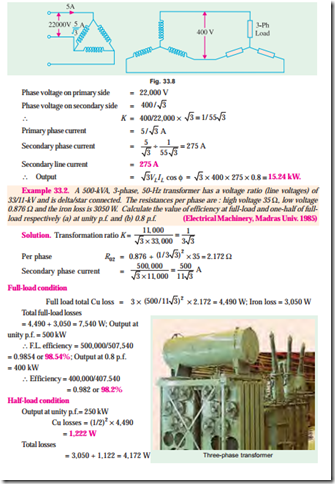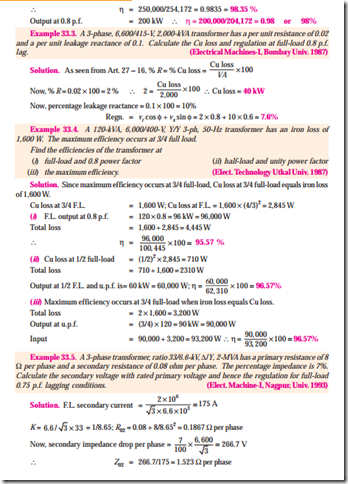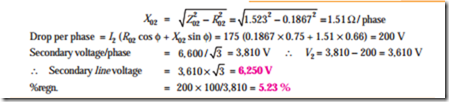Example 33.6. A 100-kVA, 3-phase, 50-Hz 3,300/400 V transformer is D-connected on the h.v. side and Y-connected on the l.v. side. The resistance of the h.v. winding is 3.5 W per phase and that of the l.v. winding 0.02 W per phase. Calculate the iron losses of the transformer at normal voltage and frequency if its full-load efficiency be 95.8% at 0.8 p.f. (lag).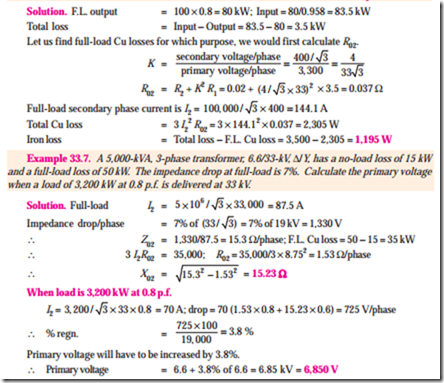Example 33.8. A 3-phase transformer has its primary connected in D and its secondary in Y. It has an equivalent resistance of 1% and an equivalent reactance of 6%. The primary applied voltage is 6,600 V. What must be the ratio of transformation in order that it will deliver 4,800 V at full-load current and 0.8 power factor (lag) ?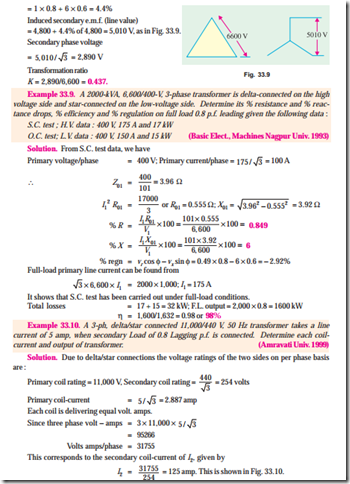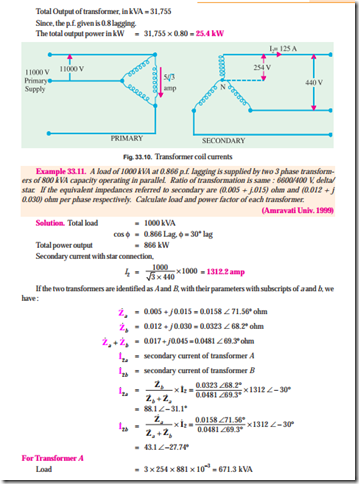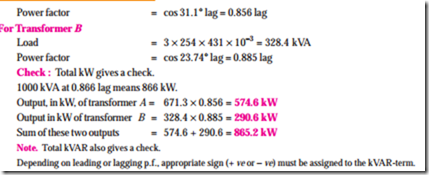Tutorial Problem No. 33.1

1. A 3-phase, star-connected alternator generates 6,360 V per phase and supplies 500 kW at a p.f. 0.9 lagging to a load through a step-down transformer of turns 40 : 1. The transformer is delta connected on the primary side and star-connected on the secondary side. Calculate the value of the line volts at the load. Calculate also the currents in (a) alternator windings (b) transformer primary windings (c) transformen secondary windings.

[476 V (a) 29.1 A (b) 16.8 A (c) 672 A]

2. A 11,000/6,600 V, 3-f, transformer has a star-connected primary and a delta-connected secondary. It supplies a 6.6 kV motor having a star-connected stator, developing 969.8 kW at a power factor of 0.9 lagging and an efficiency of 92 per cent. Calculate (i) motor line and phase currents (ii) transformer secondary current and (iii) transformer primary current.

[(a) Motor ; IL = Iph = 126.3 A (b) phase current 73 A (c) 75.8 A]